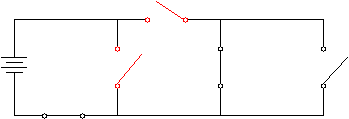Understanding Power Supplies and Simple Circuits

Key Terms

• Power supply
• Electrical circuit
• Switch
• Closed circuit
• Open circuit

Objectives

• Recognize the function and representation of a simple power supply
• Analyze a simple electrical circuit
• Identify the function of switches in a circuit
Electronic devices operate by applying voltages that create electric currents through various components. These currents can perform a number of functions: for example, they create heat on an electric range (stove), they create light in a light bulb, and they carry information from point to point in a processor. So, how do we get a voltage so we can perform these functions? The answer is what we can broadly call power supplies.

Please note: do not attempt to replicate the diagrams in this article. Doing so may result in electric shock, injury, or death. These examples are provided only for theoretical discussion and not for actual/physical use.

Power Supplies

An electric power supply is a device or system that converts some form of energy into electrical energy. For example, a battery converts chemical energy to electrical energy by way of chemical reactions that create a voltage across the two terminals (one of which is labeled "+" and the other "-"). In the case of your electric utility company, the power plant burns coal or uses nuclear fuel to turn a turbine that, through the use of magnets, generates a voltage that power lines carry to your home. Solar panels convert the energy in light to electrical energy.

Whatever the source, a power supply converts some form of stored or otherwise available energy into electrical energy. (According to a fundamental tenet of physics, energy is neither created nor destroyed--it can only change forms.) But what does a power supply look like with regard to our discussion of voltage and current? Below is an illustration of a simple power supply with a positive terminal and negative terminal. The positive terminal has a net positive charge, and the negative terminal has a net negative charge. We'll call the negative terminal ground.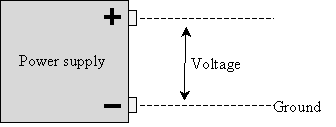Because of the excess positive charge at the positive terminal and the excess negative charge at the negative terminal, a positive charge will be repelled from the positive terminal and attracted toward the negative terminal.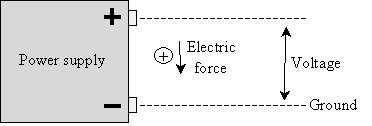For illustration purposes, let's consider a 1.5V battery--this is the potential energy difference between the two battery terminals per coulomb of charge. We'll still call the negative terminal ground, because a positive charge will "fall" away from the positive terminal toward the negative terminal, as shown above. In the diagram below, we simply assume that the battery is surrounded by air, which is an insulator (it doesn't conduct charge).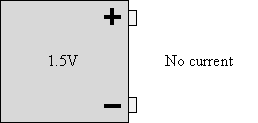But what if we connect a conducting material-such as copper wire-across the terminals of the battery? We then have both a potential difference between the two terminals and a pathway for charge to travel. As a result, current will flow from the positive terminal to the negative terminal.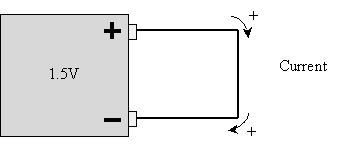Interested in learning more? Why not take an online Electronics course?

At this point, you may be a little confused as to why we are showing the flow of positive charge. Recall that conductors allow the free flow of loosely bound electrons--thus, we would expect that what would happen is that negative charge would flow from the negative terminal (where it is in excess) toward the positive terminal (to balance the positive charge there). This is actually what happens, but by historical accident, positive charge was associated with protons rather than electrons (the charge of electrons could just have legitimately been called positive). As it turns out, a given flow of positive charge in one direction is equivalent to the same flow of negative charge in the opposite direction.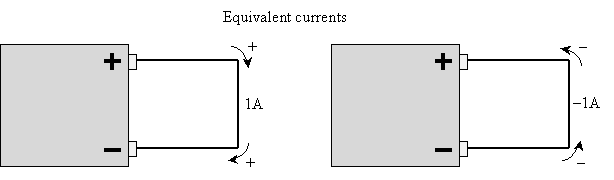But to align our study with the conventions of physics, we'll generally talk about positive current--that is, positive charge flowing from a higher voltage (the positive terminal) to a lower voltage (ground). Incidentally, you shouldn't connect just a wire or other good conductor to a battery in this manner--it will very quickly exhaust the battery.

A Simple Electric Circuit

What we see above, where the two terminals of a power supply (battery, for example) are connected to each other, is a simple electrical circuit. An electrical circuit is, as you can probably judge from the example above and the name, a closed loop through which current can flow. Because the circuit above contains no other components besides the battery, however, it's not a very interesting example.

Note that electrons can only flow from one point in a material (or combination of materials) to another if there is a continuous path through conducting material (a conductor) between the points. In the simple circuit above, such a path exists between the battery terminals. But what if we introduce a break in the wire? Then, of course, current will not flow. If we can "break" and "unbreak" the circuit at will, then we can turn on and off the flow of charge: in other words, we've introduced a switch into the circuit. Note that when the switch is closed (connecting the wire), the configuration is called a closed circuit. When the switch is open, it is called an open circuit.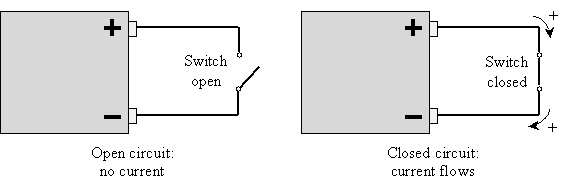Finally, let's replace our bulky battery with a more conventional symbol for a power supply--one that you'll commonly see in real-world diagrams of electrical circuits.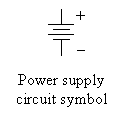Note that the positive terminal is on the side with the longer horizontal bar; the negative terminal is on the side with the shorter bar. Both are labeled above, but these labels are generally not shown. Thus, our simple switch circuit looks like the following.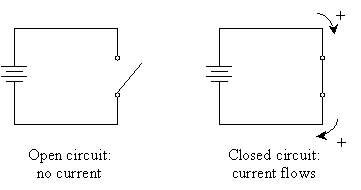Thus, we have taken the first step into the world of electrical circuits. Again, even with the switch, this circuit is not all that interesting: all it does is quickly deplete the energy stored in the battery when the circuit is closed. The important point to note, however, is that by "breaking" the circuit, we can control whether current is allowed to flow. This useful approach allows us to turn lights on and off at wall switches, for instance.

Practice Problem: Determine which way current will flow in the simple closed circuit shown below.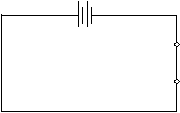Solution: We learned that, by convention, we interpret current as the flow of positive charge from the positive (positively charged) terminal to the negative (negatively charged) terminal. The battery in the simple circuit above is oriented such that the positive terminal is on the left. Thus, current will flow in the counterclockwise direction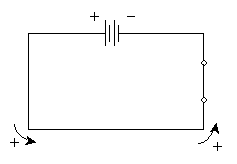Practice Problem: Will any current flow in the circuit below? Why or why not?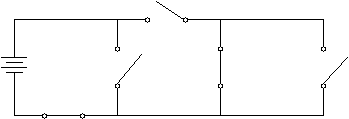Solution: Although this circuit is a little more complicated than the simple circuits we have seen thus far, we can apply the same principles we have already used. Remember that current only flows when there is a conducting path from a higher voltage (the positive terminal of the power supply) to a lower voltage (or ground-the negative terminal). In this case, note that the switches highlighted below are both open, giving the current no way to reach the negative terminal. Thus, no current flows in this circuit.